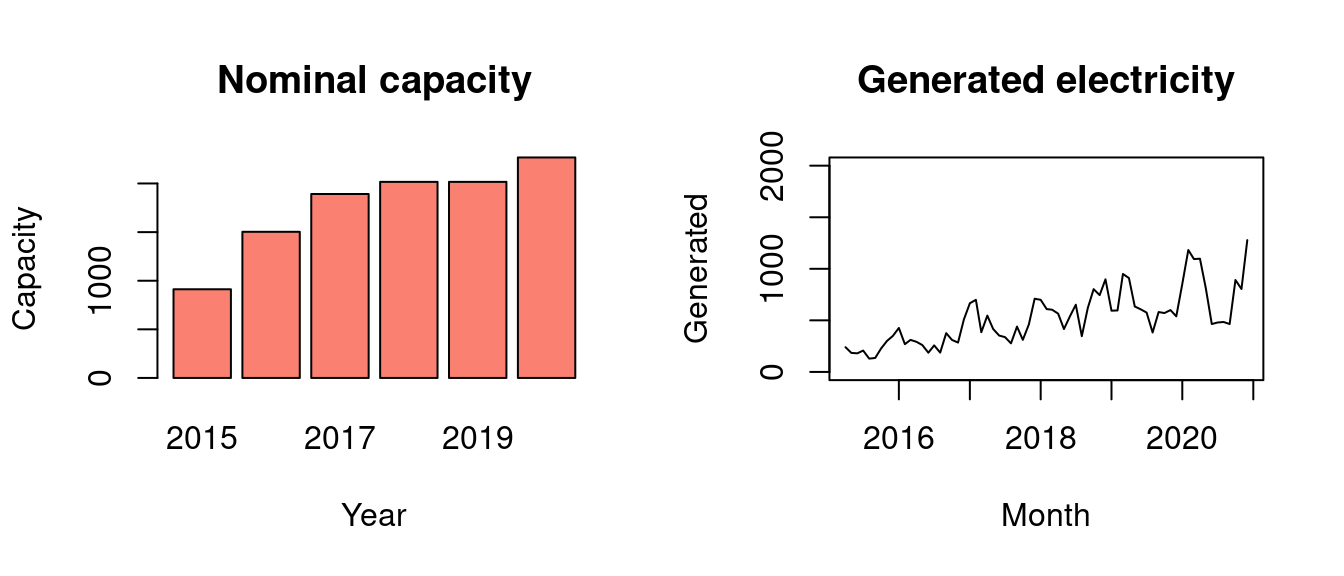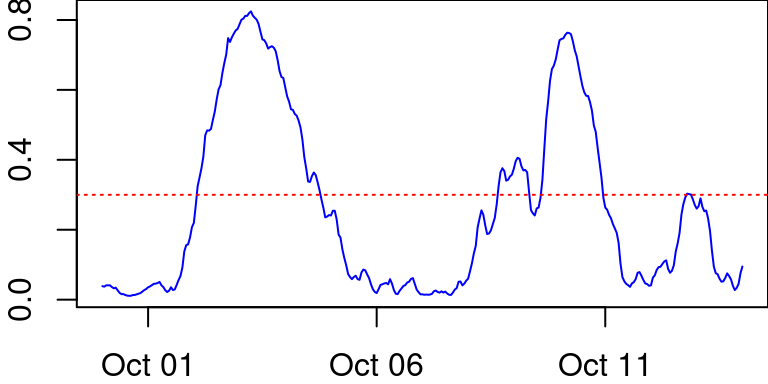# 1 Introduction

Wind power plays an important role when we try to find solutions for the climate crisis.

Carbon neutrality requires a huge number of new wind power plants in Finland. According to Motiva by the end of 2019 there were 754 wind turbines in Finland, generating about 5.9 TWh electricity. That amounts to 7% of domestic consumption.

If the electricity generation pattern of wind turbines is properly understood, it is possible to dynamically vary consumption accordingly. Thus the intermittent nature of wind power generation would be manageable without additional polluting and expensive reserve power systems.

## 1.1 Generation Capacity

If a wind turbine’s nominal capacity is 3MW, it could generate $$3MW \times 24h = 72MWh$$ in a day. However, such a turbine in Finland generates about a third of that (Tuulivoimayhdistys, 2020). Generation depends heavily on weather conditions and fluctuates according to for example the season, time of day, and location.

The 754 wind turbines in Finland have a total capacity of 2284MW. The efficiency is thus the yearly generation divided by nominal capacity

$\frac{5.9TWh}{2284MW \times 365 \times 24h} = 29.5\%$

## 1.2 Problem Analysis

What is the trend of the generation efficiency? What kind of generation patterns should we prepare for, when more and more electricity is generated by wind turbines?

Let’s fit a linear regression to a time series of wind generation values. The regression helps to understand both the potential capacity and mean of wind power. The mean, and the trend, show us what kind of real efficiency to expect from wind generation in Finland. The trend can be used to make rough estimate of the potential: For example, are all the good locations already taken or is the trend actually rising? A rising, or even a constant, trend would imply that there’s still profitable untapped potential.

## 1.3 Modeling Idea

Fit a simple linear regression model to the data. Compare the simple fit with another, hierarchical model that makes use of seasonal information.

The generation efficiency varies according to weather. But instead of gathering arbitrary weather data, let’s use something more generalizable. In Finland the weather varies according to season. From Wikipedia/Suomen_ilmasto and Ilmatieteenlaitos/termiset-vuodenajat:

• During the winter, the mean temperature is below zero.
• In the summer, the mean temperature is above +10°C.

Most of the wind farms are in the north-western coast, so let’s use seasonal data from around Oulu region.

• On average, summer begins Jun 1st and ends Sep 1st (3 months)
• Winter begins Nov 1st and ends Apr 1st (5 months)
• Spring and autumns last 2 months each.

# 2 Description of Data

library("ggplot2")
library("bayesplot")
library("data.table")
library("lubridate")
library("dplyr")
library("rstan")
library("bayesplot")
library("loo")
d <- fread("~/notebooks/project/fingrid_10y.csv")

We’ll use open data from the Finnish transmission system operator Fingrid. This is not a ready-made data set, so it hasn’t been used before as such. There are, of course, analysis of other wind generation data, for example (VTT, 2013), (Koivisto et al., 2014), and (Koivisto, 2015).

The data represents hourly wind power generation from March 2015 to November 2020.

par(mfrow=c(1, 2))
d %>% group_by(Year = year(timestamp)) %>%
summarise(Capacity = last(capacity), .groups = 'drop') %>%
with(barplot(Capacity ~ Year, col = 'salmon', main = "Nominal capacity"))
d %>% group_by(Month = ceiling_date(timestamp, 'month')) %>%
summarise(Generated = mean(generated), .groups = 'drop') %>%
with(plot(Generated ~ Month, type = 'l', main = 'Generated electricity',
ylim = c(0, 2000)))During the past six years the wind power generation capacity in Finland has more than tripled.

c(first(d$capacity), last(d$capacity), last(d$capacity) / first(d$capacity))
##   625.0000 2268.0000    3.6288

We’ll use the relative efficiency (generation divided by nominal capacity) to see how the generation efficiency has developed over years.

## 2.1 Wide Fluctations

The data shows the intermittent nature of wind. The generated power fluctuates wildly around the year. For example, here’s a two week time span showing how the generated power quickly drops from near maximum to near minimum. The horizontal line shows the average power generation efficiency.

par(mar=c(2, 2, 0, 0))
d %>% filter(timestamp %within% as.interval(weeks(2), ymd("2017-09-30"))) %>%
with(plot(geneff ~ timestamp, type='l', col='blue', lwd=1))
abline(h=.3, col='red', lty=3)## 2.2 Weekly Summary

We’ll summarize the 50k rows of hourly observations to weekly mean and standard deviation to make modeling feasible. Summarizing smooths the data while retaining information about the extent of fluctuations. There are 298 weeks of data.

# Weekly time series
w <- mutate(d, week = isoweek(timestamp), month = month(timestamp)) %>%
group_by(ts = floor_date(timestamp, "week")) %>%
summarise(mug = mean(geneff), sig = sd(geneff), month = median(month),
week = median(week), .groups = 'drop')
nrow(w)
##  298
w$ma12 <- frollmean(x=w$mug, n=12, align = "center") # 12 weeks moving average
ggw <- ggplot(data = w, aes(x=ts, y=mug)) +
geom_point(color = "#56B4E988", size = 1) +
labs(x = "Week", y = "Generation efficiency")
ggw + geom_line(data = w, aes(y = ma12), color = "#0072B288", na.rm = TRUE) +
labs(title = "Weekly efficiency and 12 weeks moving average")